MOTIONOFBODIES MOTION OF BODIES

General

The study of the relationship between the motion of bodies or objects and the forces acting on them is often called the study of "force and motion." In a more specific sense, the relationship between velocity, acceleration, and distance is known as kinematics.

Uniform Motion

Motion may be defined as a continuing change of position or place, or as the process in which a body undergoes displacement. When an object is at different points in space at different times, that object is said to be in motion, and if the distance the object moves remains the same for a given period of time, the motion may be described as uniform. Thus, an object in uniform motion always has a constant speed.

Speed and Velocity

In everyday usage, speed and velocity often mean the same thing. In physics they have definite and distinct meanings. Speed refers to how fast an object is moving, or how far the object will travel in a specific time. The speed of an object tells nothing about the direction an object is moving. For example, if the information is supplied that an airplane leaves New York City and travels 8 hours at a speed of 150 mph, this information tells nothing about the direction in which the airplane is moving. At the end of 8 hours, it might be in Kansas City, or if it traveled in a circular route, it could be back in New York City.

Velocity is that quantity in physics which denotes both the speed of an object and the direction in which the object moves. Velocity can be defined as the rate of motion in a particular direction.

The average velocity of an object can be calculated using the formula,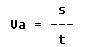where:

Va = the average velocity.
s = the rate of motion or average speed.
t = the elapsed time.

Acceleration

Acceleration is defined by the physicist as the rate of change of velocity. If the velocity of an object is increased from 20 mph to 30 mph, the object has been accelerated. If the increase in velocity is 10 mph in 5 seconds, the rate of change in velocity is 10 mph in 5 seconds, or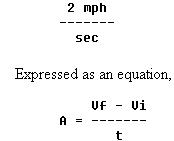where:

A = acceleration.
Vf = the final velocity (30 mph).
Vi = the initial velocity (20 mph).
t = the elapsed time.

The example used can be expressed as follows: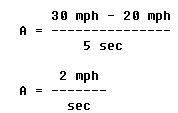If the object accelerated to 22 mph in the first second, 24 mph in the next second, and 26 mph in the third second, the change in velocity each second is 2 mph. The acceleration is said to be constant, and the motion is described as uniformly accelerated motion.

If a body has a velocity of 3 mph at the end of the first second of its motion, 5 mph at the end of the next second, and 8 mph at the end of the third second, its motion is described as acceleration, but it is variable accelerated motion.

Newton's Law of Motion

When a magician snatches a tablecloth from a table and leaves a full setting of dishes undisturbed, he is not displaying a mystic art; he is demonstrating the principle of inertia.

Inertia is responsible for the discomfort felt when an airplane is brought to a sudden halt in the parking area and the passengers are thrown forward in their seats. Inertia is a property of matter. This property of matter is described by Newton's first law of motion, which states:

Objects at rest tend to remain at rest; objects in motion tend to remain in motion at the same speed and in the same direction.

Bodies in motion have the property called momentum. A body that has great momentum has a strong tendency to remain in motion and is therefore hard to stop. For example, a train moving at even low velocity is difficult to stop because of its large mass. Newton's second law applies to this property. It states:

When a force acts upon a body, the momentum of that body is changed. The rate of change of momentum is proportional to the applied force.

The momentum of a body is defined as the product of its mass and its velocity. Thus,

Momentum = mass x velocity  or,  M = mV

Now if a force is applied, the momentum changes at a rate equal to the force or:   F = rate of change of momentum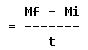Substituting mV for M: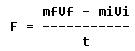Since the mass does not usually change, mf = mi = m. Then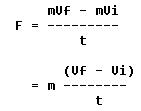From the previous section the second term is recognized as acceleration. Then the second law becomes:   F = ma

On earth, gravity exerts a force on each body causing an acceleration of 32 ft/sec2 which is usually designated as "g". The force is commonly called weight, W. Using the formula above:

W = mg

and;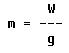Then on earth the second law becomes: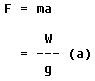The following examples illustrate the use of this formula.

Example: A train weighs 32,000 lbs and is traveling at 10 ft/sec. What force is required to bring it to rest in 10 seconds?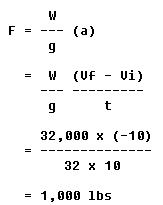The negative sign means that the force must be applied against the train's motion.

Example: An aircraft weighs 6,400 pounds. How much force is needed to give it an acceleration of 6 ft/sec2 ?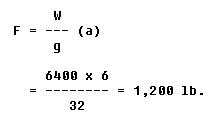Newton's third law of motion is often called the law of action and reaction. It states that for every action there is an equal and opposite reaction. This means that if a force is applied to an object, the object will supply a resistive force exactly equally to and in the opposite direction of the force applied. It is easy to see how this might apply to objects at rest. For example, as a man stands on the floor, the floor exerts a force against his feet exactly equal to his weight. But this law is also applicable when a force is applied to an object in motion.

When a force applied to an object is more than sufficient to produce and sustain uniform motion, inertia of the object will cause such a resistive force that the force opposing the motion of the object equals the force producing the motion. This resistance to change in velocity due to inertia is usually referred to as internal force. When several forces act upon an object to produce accelerated motion, the sums of the external forces are in a state of unbalance; however, the sums of the external and the internal forces are always in a state of balance, whether motion is being either sustained or produced.

Forces always occur in pairs. The term "acting force" means the force one body exerts on a second body, and reacting force means the force the second body exerts on the first.

When an aircraft propeller pushes a stream of air backward with a force of 500 pounds, the air pushes the blades forward with a force of 500 pounds. This forward force causes the aircraft to move forward. In like manner, the discharge of exhaust gases from the tailpipe of a turbine engine is the action which causes the aircraft to move forward.

The three laws of motion which have been discussed here are closely related. In many cases, all three laws may be operating on a body at the same time.

Circular Motion

 Circular motion is the motion of an object along a curved path that has a constant radius. For example, if one end of a string is tied to an object and the other end is held in the hand, the object can be swung in a circle. The object is constantly deflected from a straight (linear) path by the pull exerted on the string, as shown in figure 7-38. As an object in figure 7-38 travels along the circumference from X to Y, the pull or force on the string deflects it from Y toward Z. This pull is called centripetal force, which deflects an object from a straight path and forces it to travel in a curved path.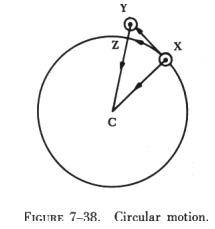Thus, the string exerts a centripetal force on the object, and the object exerts an equal but opposite force on the string, obeying Newton's third law of motion.

The force that is equal to centripetal force, but acting in an opposite direction, is called centrifugal force. In the example of figure 7-38, it is the force exerted by the object on the string. Without a centripetal force, there is no centrifugal force.

Centripetal force is always directly proportional to the mass of the object in circular motion. Thus, if the mass of the object in figure 7-38 is doubled, the pull on the string must be doubled to keep the object in its circular path, provided the speed of the object remains constant.

Centripetal force is inversely proportional to the radius of the circle in which an object travels. If the string in figure 7-38 is shortened and the speed remains constant, the pull on the string must be increased since the radius is decreased, and the string must pull the object from its linear path more rapidly.

Using the same reasoning, the pull on the string must be increased if the object is swung more rapidly in its orbit. Centripetal force is thus directly proportional to the square of the velocity of the object. The formula for centripetal force is: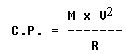where:

M = the mass of the object.
V = velocity.
R = radius of the object's path.

Rotary Motion

The motion of a body turning about an axis is called rotary motion. This is the familiar motion that occurs as the crankshaft of an engine turns.

The difference between rotary and circular motion is that, in the case of rotary motion, the body or object spins, while in circular motion the whole object moves along a curved path.

When an object spins at a constant speed about a fixed axis, it has uniform rotary motion. When its direction or rate of spin changes, it has variable rotary motion.

Momentum

Momentum is defined as the product of an object's mass and its velocity. The force required to accelerate an object is proportional to an object's mass and the acceleration given it. Acceleration has been defined as the rate of change of an object's velocity. This is expressed as a formula: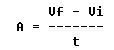where:

A = acceleration.
Vf = final velocity.
Vi = initial velocity.
t = elapsed time.

Newton's second law of motion, F = MA, involves acceleration. If the original expression for acceleration is substituted in Newton's second law, it becomes: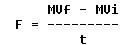This formula can be further resolved to illustrate momentum by multiplying both sides by t:

Ft = MVf - MVi

This formula illustrates that an object's momentum is a product of its mass and its velocity.